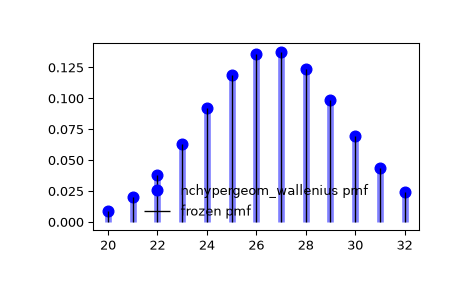# scipy.stats.nchypergeom_wallenius¶

scipy.stats.nchypergeom_wallenius = <scipy.stats._discrete_distns.nchypergeom_wallenius_gen object>[source]

A Wallenius’ noncentral hypergeometric discrete random variable.

Wallenius’ noncentral hypergeometric distribution models drawing objects of two types from a bin. M is the total number of objects, n is the number of Type I objects, and odds is the odds ratio: the odds of selecting a Type I object rather than a Type II object when there is only one object of each type. The random variate represents the number of Type I objects drawn if we draw a pre-determined N objects from a bin one by one.

As an instance of the rv_discrete class, nchypergeom_wallenius object inherits from it a collection of generic methods (see below for the full list), and completes them with details specific for this particular distribution.

Notes

Let mathematical symbols $$N$$, $$n$$, and $$M$$ correspond with parameters N, n, and M (respectively) as defined above.

The probability mass function is defined as

$p(x; N, n, M) = \binom{n}{x} \binom{M - n}{N-x} \int_0^1 \left(1-t^{\omega/D}\right)^x\left(1-t^{1/D}\right)^{N-x} dt$

for $$x \in [x_l, x_u]$$, $$M \in {\mathbb N}$$, $$n \in [0, M]$$, $$N \in [0, M]$$, $$\omega > 0$$, where $$x_l = \max(0, N - (M - n))$$, $$x_u = \min(N, n)$$,

$D = \omega(n - x) + ((M - n)-(N-x)),$

and the binomial coefficients are defined as

$\binom{n}{k} \equiv \frac{n!}{k! (n - k)!}.$

nchypergeom_wallenius uses the BiasedUrn package by Agner Fog with permission for it to be distributed under SciPy’s license.

The symbols used to denote the shape parameters (N, n, and M) are not universally accepted; they are chosen for consistency with hypergeom.

Note that Wallenius’ noncentral hypergeometric distribution is distinct from Fisher’s noncentral hypergeometric distribution, which models take a handful of objects from the bin at once, finding out afterwards that N objects were taken. When the odds ratio is unity, however, both distributions reduce to the ordinary hypergeometric distribution.

The probability mass function above is defined in the “standardized” form. To shift distribution use the loc parameter. Specifically, nchypergeom_wallenius.pmf(k, M, n, N, odds, loc) is identically equivalent to nchypergeom_wallenius.pmf(k - loc, M, n, N, odds).

References

1

Agner Fog, “Biased Urn Theory”. https://cran.r-project.org/web/packages/BiasedUrn/vignettes/UrnTheory.pdf

2

“Wallenius’ noncentral hypergeometric distribution”, Wikipedia, https://en.wikipedia.org/wiki/Wallenius’_noncentral_hypergeometric_distribution

Examples

>>> from scipy.stats import nchypergeom_wallenius
>>> import matplotlib.pyplot as plt
>>> fig, ax = plt.subplots(1, 1)


Calculate the first four moments:

>>> M, n, N, odds = 140, 80, 60, 0.5
>>> mean, var, skew, kurt = nchypergeom_wallenius.stats(M, n, N, odds, moments='mvsk')


Display the probability mass function (pmf):

>>> x = np.arange(nchypergeom_wallenius.ppf(0.01, M, n, N, odds),
...               nchypergeom_wallenius.ppf(0.99, M, n, N, odds))
>>> ax.plot(x, nchypergeom_wallenius.pmf(x, M, n, N, odds), 'bo', ms=8, label='nchypergeom_wallenius pmf')
>>> ax.vlines(x, 0, nchypergeom_wallenius.pmf(x, M, n, N, odds), colors='b', lw=5, alpha=0.5)


Alternatively, the distribution object can be called (as a function) to fix the shape and location. This returns a “frozen” RV object holding the given parameters fixed.

Freeze the distribution and display the frozen pmf:

>>> rv = nchypergeom_wallenius(M, n, N, odds)
>>> ax.vlines(x, 0, rv.pmf(x), colors='k', linestyles='-', lw=1,
...         label='frozen pmf')
>>> ax.legend(loc='best', frameon=False)
>>> plt.show()Check accuracy of cdf and ppf:

>>> prob = nchypergeom_wallenius.cdf(x, M, n, N, odds)
>>> np.allclose(x, nchypergeom_wallenius.ppf(prob, M, n, N, odds))
True


Generate random numbers:

>>> r = nchypergeom_wallenius.rvs(M, n, N, odds, size=1000)


Methods

 rvs(M, n, N, odds, loc=0, size=1, random_state=None) Random variates. pmf(k, M, n, N, odds, loc=0) Probability mass function. logpmf(k, M, n, N, odds, loc=0) Log of the probability mass function. cdf(k, M, n, N, odds, loc=0) Cumulative distribution function. logcdf(k, M, n, N, odds, loc=0) Log of the cumulative distribution function. sf(k, M, n, N, odds, loc=0) Survival function (also defined as 1 - cdf, but sf is sometimes more accurate). logsf(k, M, n, N, odds, loc=0) Log of the survival function. ppf(q, M, n, N, odds, loc=0) Percent point function (inverse of cdf — percentiles). isf(q, M, n, N, odds, loc=0) Inverse survival function (inverse of sf). stats(M, n, N, odds, loc=0, moments=’mv’) Mean(‘m’), variance(‘v’), skew(‘s’), and/or kurtosis(‘k’). entropy(M, n, N, odds, loc=0) (Differential) entropy of the RV. expect(func, args=(M, n, N, odds), loc=0, lb=None, ub=None, conditional=False) Expected value of a function (of one argument) with respect to the distribution. median(M, n, N, odds, loc=0) Median of the distribution. mean(M, n, N, odds, loc=0) Mean of the distribution. var(M, n, N, odds, loc=0) Variance of the distribution. std(M, n, N, odds, loc=0) Standard deviation of the distribution. interval(alpha, M, n, N, odds, loc=0) Endpoints of the range that contains fraction alpha [0, 1] of the distribution